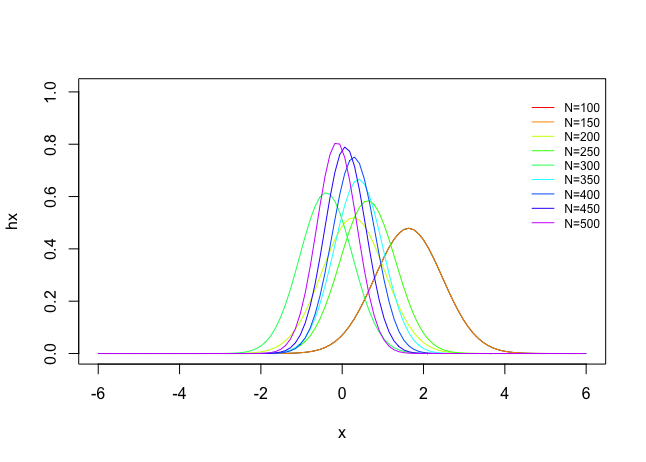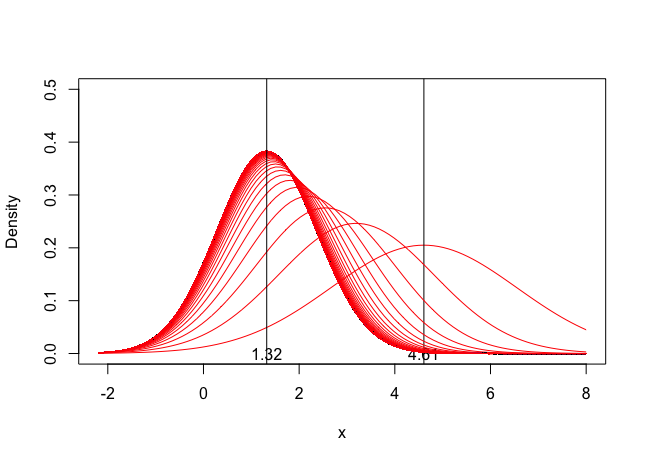# Normal Likelihood with Normal Prior

Often, it is useful to simulate the Bayesian updating process, to study how the posterior changes with the sample moments of $$x$$ . Therefore I have written a R function, which takes a vector of normally distributed data $$x$$ , a prior (the hyper-parameters) mean $$\mu$$ and variance $$\tau^2$$ , and then calculates the posterior.

# Examples

## Example 1: Basic usage

For instance, suppose 10 observations are coming from $N(\theta,100)$ . Assume that the prior on $$\theta$$ is $$N(20,20)$$ . Using the numerical example in the R code below, the posterior is $$N(1.322028, 1.086328)$$. These three densities are shown in Figure 1.# Example 2: Sample Size Simulation

You can also easily make a sample size Monte Carlo simulation## Example 3: Recursive Bayesian Updating

Simulate the updating process for recursive Bayesian updatingTags:

Updated: